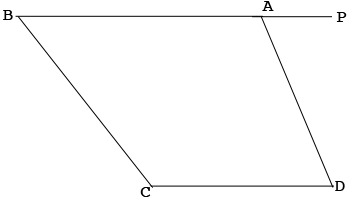SEARCH HOMEMath Central Quandaries & QueriesQuestion from Gautham: In trapezoid ABCD, sides AB and CD are parallel, angle A = 2angle D, and angle C = 3angle B. Find angle A.Hi,

I drew a diagram of your trapezoid and extended $BA$ to a point $P.$Since $AB$ and $DC$ are parallel what is the relationship between the interior angle at $D$ and the angle $PAD?$

Penny* Registered trade mark of Imperial Oil Limited. Used under license.Math Central is supported by the University of Regina and the Imperial Oil Foundation.